# Conjugate Heat Transfer

January 6, 2014

In this blog post we will explain the concept of conjugate heat transfer and show you some of its applications. Conjugate heat transfer corresponds with the combination of heat transfer in solids and heat transfer in fluids. In solids, conduction often dominates whereas in fluids, convection usually dominates. Conjugate heat transfer is observed in many situations. For example, heat sinks are optimized to combine heat transfer by conduction in the heat sink with the convection in the surrounding fluid.

### Heat Transfer by Solids and Fluids

#### Heat Transfer in a Solid

In most cases, heat transfer in solids, if only due to conduction, is described by Fourier’s law defining the conductive heat flux, q, proportional to the temperature gradient: q=-k\nabla T.

For a time-dependent problem, the temperature field in an immobile solid verifies the following form of the heat equation:

\rho C_{p} \frac{\partial T}{\partial t}=\nabla \cdot (k\nabla T) +Q

#### Heat Transfer in a Fluid

Due to the fluid motion, three contributions to the heat equation are included:

1. The transport of fluid implies energy transport too, which appears in the heat equation as the convective contribution. Depending on the thermal properties on the fluid and on the flow regime, either the convective or the conductive heat transfer can dominate.
2. The viscous effects of the fluid flow produce fluid heating. This term is often neglected, nevertheless, its contribution is noticeable for fast flow in viscous fluids.
3. As soon as a fluid density is temperature-dependent, a pressure work term contributes to the heat equation. This accounts for the well-known effect that, for example, compressing air produces heat.

Accounting for these contributions, in addition to conduction, results in the following transient heat equation for the temperature field in a fluid:

\rho C_{p} \frac{\partial T}{\partial t}+\rho C_p\bold{u}\cdot\nabla T= \alpha_p {T}\left( \frac{\partial p_\mathrm{A}}{\partial t}+\bold{u}\cdot\nabla p_\mathrm{A}\right)+\tau : S+\nabla \cdot (k\nabla T) +Q

### Conjugate Heat Transfer Applications

#### Effective Heat Transfer

Efficiently combining heat transfer in fluids and solids is the key to designing effective coolers, heaters, or heat exchangers.

The fluid usually plays the role of energy carrier on large distances. Forced convection is the most common way to achieve high heat transfer rate. In some applications, the performances are further improved by combining convection with phase change (for example liquid water to vapor phase change).

Even so, solids are also needed, in particular to separate fluids in a heat exchanger so that fluids exchange energy without being mixed.Flow and temperature field in a shell-and-tube heat exchanger illustrating heat transfer between two fluids separated by the thin metallic wall.

Heat sinks are usually made of metal with high thermal conductivity (e.g. copper or aluminum). They dissipate heat by increasing the exchange area between the solid part and the surrounding fluid.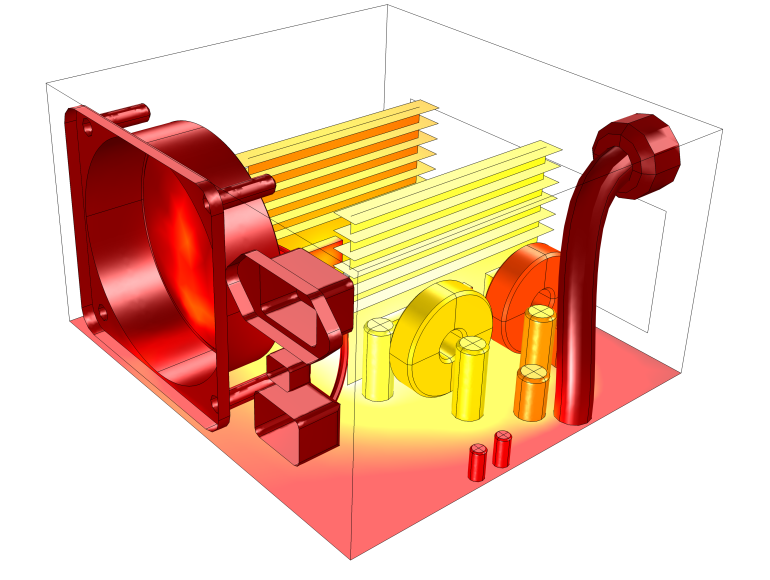Temperature field in a power supply unit cooling due to an air flow generated by an extracting fan and a perforated grille. Two aluminum fins are used to increase the exchange area between the flow and the electronic components.

#### Energy Savings

Heat transfer in fluids and solids can also be combined to minimize heat losses in various devices. Because most gases (especially at low pressure) have small thermal conductivities, they can be used as thermal insulators… provided they are not in motion. In many situations, gas is preferred to other material due to its low weight. In any case, it is important to limit the heat transfer by convection, in particular by reducing the natural convection effects. Judicious positioning of walls and use of small cavities helps to control the natural convection. Applied at the micro scale, the principle leads to the insulation foam concept where tiny cavities of air (bubbles) are trapped in the foam material (e.g. polyurethane), which combines high insulation performances with light weight.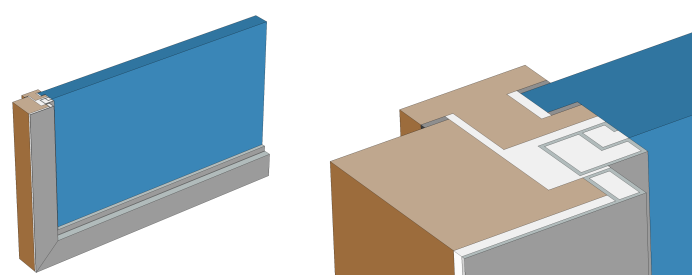Window cross section (left) and zoom-in on the window frame (right).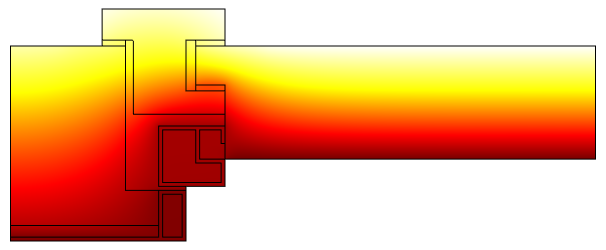Temperature profile in a window frame and glazing cross section from ISO 10077-2:2012 (thermal performance of windows).

### Fluid and Solid Interactions

#### Fluid/Solid Interface

The temperature field and the heat flux are continuous at the fluid/solid interface. However, the temperature field can rapidly vary in a fluid in motion: close to the solid, the fluid temperature is close to the solid temperature, and far from the interface, the fluid temperature is close to the inlet or ambient fluid temperature. The distance where the fluid temperature varies from the solid temperature to the fluid bulk temperature is called the thermal boundary layer. The thermal boundary layer size and the momentum boundary layer relative size is reflected by the Prandtl number (Pr=C_p \mu/k): for the Prandtl number to equal 1, thermal and momentum boundary layer thicknesses need to be the same. A thicker momentum layer would result in a Prandtl number larger than 1. Conversely, a Prandtl number smaller than 1 would indicate that the momentum boundary layer is thinner than the thermal boundary layer. The Prandtl number for air at atmospheric pressure and at 20°C is 0.7. That is because for air, the momentum and thermal boundary layer have similar size, while the momentum boundary layer is slightly thinner than the thermal boundary layer. For water at 20°C, the Prandtl number is about 7. So, in water, the temperature changes close to a wall are sharper than the velocity change.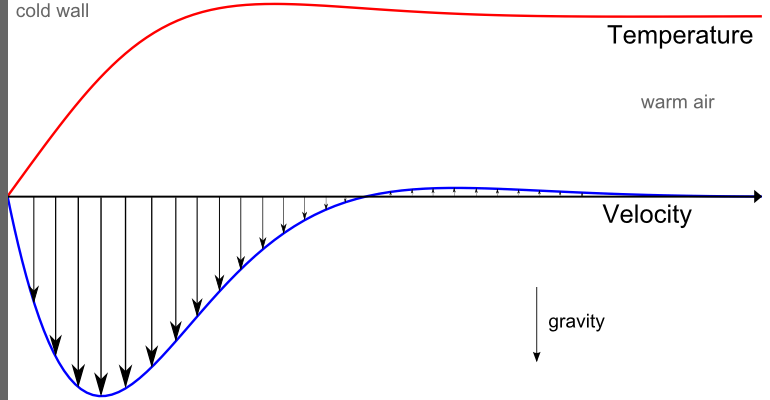Normalized temperature (red) and velocity (blue) profile for natural convection of air close to a cold solid wall.

#### Natural Convection

The natural convection regime corresponds to configurations where the flow is driven by buoyancy effects. Depending on the expected thermal performance, the natural convection can be beneficial (e.g. cooling application) or negative (e.g. natural convection in insulation layer).

The Rayleigh number, noted as Ra, is used to characterized the flow regime induced by the natural convection and the resulting heat transfer. The Rayleigh number is defined from fluid material properties, a typical cavity size, L, and the temperature difference,\Delta T, usually set by the solids surrounding the fluid:

Ra=\frac{\rho^2g\alpha_p C_p}{\mu k}\Delta T L^3

The Grashof number is another flow regime indicator giving the ratio of buoyant to viscous forces:

Gr=\frac{\rho^2g\alpha_p}{\mu^2}\Delta T L^3

The Rayleigh number can be expressed in terms of the Prandtl and the Grashof numbers through the relation Ra=Pr Gr.

When the Rayleigh number is small (typically <103), the convection is negligible and most of the heat transfer occurs by conduction in the fluid.

For a larger Rayleigh number, heat transfer by convection has to be considered. When buoyancy forces are large compared to viscous forces, the regime is turbulent, otherwise it is laminar. The transition between these two regimes is indicated by the critical order of the Grashof number, which is 109. The thermal boundary layer, giving the typical distance for temperature transition between the solid wall and the fluid bulk, can be approximated by \delta_\mathrm{T} \approx \frac{L}{\sqrt[4\,]{Ra}} when Pr is of order 1 or greater.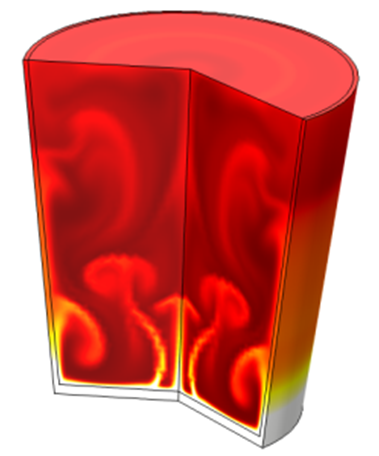Temperature profile induced by natural convection in a glass of cold water in contact with a hot surface .

#### Forced Convection

The forced convection regime corresponds to configurations where the flow is driven by external phenomena (e.g. wind) or devices (e.g. fans, pumps) that dominate buoyancy effects.

In this case the flow regime can be characterized, similarly to isothermal flow, using the Reynolds number as an indicator,Re= \frac{\rho U L}{\mu}. The Reynolds number represents the ratio of inertial to viscous forces. At low Reynolds numbers, viscous forces dominate and laminar flow is observed. At high Reynolds numbers, the damping in the system is very low, giving small disturbances. If the Reynolds number is high enough, the flow field eventually ends up in turbulent regime.

The momentum boundary layer thickness can be evaluated, using the Reynolds number, by \delta_\mathrm{M} \approx \frac{L}{\sqrt{Re}}.Streamlines and temperature profile around a heat sink cooling by forced convection.

### Radiative Heat Transfer

Radiative heat transfer can be combined with conductive and convective heat transfer described above.

In a majority of applications, the fluid is transparent to heat radiation and the solid is opaque. As a consequence, the heat transfer by radiation can be represented as surface-to-surface radiation transferring energy between the solid wall through transparent cavities. The radiative heat flux emitted by a diffuse gray surface is equal to \varepsilon n^2 \sigma T^4. When a surface is surrounded by bodies at a homogeneous T_\mathrm{amb}, the net radiative flux is q_\mathrm{r} = \varepsilon n^2 \sigma (T_\mathrm{amb}^4-T^4). When surrounding surfaces of different temperatures, each surface-to-surface exchange is determined by the surface’s view factors.

Nevertheless, both fluids and solids may be transparent or semitransparent. So radiation can occur in fluid and solids. In participating (or semitransparent) media, the radiation rays interact with the medium (solid or fluid) then absorb, emit, and scatter radiation.

Whereas radiative heat transfer can be neglected in applications with small temperature differences and lower emissivity, it plays a major role in applications with large temperature differences and large emissivities.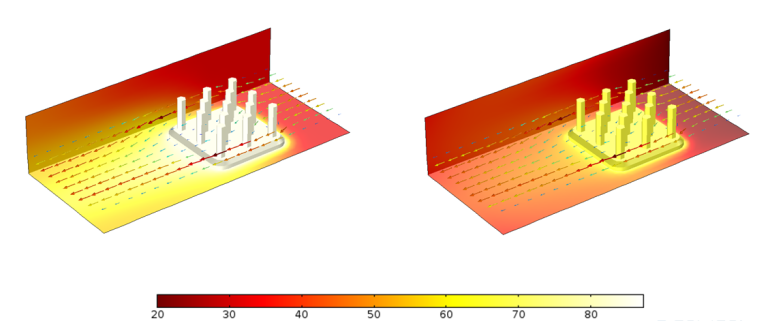Comparison of temperature profiles for a heat sink with a surface emissivity \varepsilon = 0 (left) and \varepsilon = 0.9 (right).

### Conclusion

Heat transfer in solids and heat transfer in fluids are combined in the majority of applications. This is because fluids flow around solids or between solid walls, and because solids are usually immersed in a fluid. An accurate description of heat transfer modes, material properties, flow regimes, and geometrical configurations enables the analysis of temperature fields and heat transfer. Such a description is also the starting point for a numerical simulation that can be used to predict conjugate heat transfer effects or to test different configurations in order, for example, to improve thermal performances of a given application.

### Notations

C_{p}: heat capacity at constant pressure (SI unit: J/kg/K)

g: gravity acceleration (SI unit: m/s2)

Gr: Grashof number (dimensionless number)

k: thermal conductivity (SI unit: W/m/K)

L: characteristic dimension (SI unit: m)

n: refractive index (dimensionless number)

p_\mathrm{A}: absolute pressure (SI unit: Pa)

Pr: Prandtl number (dimensionless number)

q: heat flux (SI unit: W/m2)

Q: heat source (SI unit: W/m3)

Ra: Rayleigh number (dimensionless number)

S: strain rate tensor (SI unit: 1/s)

T: temperature field (SI unit:K)

T_\mathrm{amb}: ambient temperature (SI unit: K)

\bold{u}: velocity field (SI unit: m/s)

U: typical velocity magnitude (SI unit: m/s)

\alpha_{p}: thermal expansion coefficient (SI unit: 1/K)

\delta_\mathrm{M}: momentum boundary layer thickness (SI unit: m)

\delta_\mathrm{T}: thermal layer thickness (SI unit: m)

\Delta T: characteristic temperature difference (SI unit: K)

\varepsilon: surface emissivity (dimensionless number)

\rho: density (SI unit: kg/m3)

\sigma: Stefan-Boltzmann constant (SI unit: W/m2T4)

\tau: viscous stress tensor (SI unit: N/m2)

#### Categories##### ali hassan
January 15, 2014

How can using conjugate heat transfer model in case flow boiling water through porous media ?##### Nicolas Huc
January 20, 2014

Conjugate heat transfer interface in COMSOL Multiphysics is dedicated to heat transfer in solids and non-isothermal flow for free flows. If the model scale is small enough (so that the porous cavities are explicitly represented), this interface may be used using a similar approach as shown in http://www.comsol.com/model/boiling-water-3972 .
Otherwise a porous media flow model should be used instead of the free flow model.##### Ana Tostao
July 16, 2014

Can I use Conjugate HT interface to simulate the air flow through a porous media? There is any example in COMSOL gallery?##### Nicolas Huc
July 17, 2014

Assuming local thermal equilibrium you can model heat transfer and flow in a porous media. A simple tutorial is available on http://www.comsol.com/model/free-convection-in-porous-medium-278, it shows how to set up a flow and a heat interfaces in porous media.##### Hassanein Mobarek
November 10, 2014

Hi,
I am willing to simulate soil sample that has a water resource from the bottom and a heat source from top, is the conjugate heat transfer is the best physics or not?##### Hamla Ilyes
March 9, 2015

hi sir

I try to make a geometry using COMSOL Multiphysics software, but I can not carry it out.
I am looking for someone to give me some ideas how to use this software ready
if you want to help me, I’ll send you the dimensions of this geometry.

Thank you in advance
ilyes##### manik kumar
June 23, 2015

hey i want to what physics do i choose for fluid flow over oscillating heated cylinder time dependent…..:)##### manik kumar
June 23, 2015

hey guys i want to knoe that what physics shall i use for the fluid flow over heated cylinder for lumped analysis##### Nicolas Huc
August 13, 2015

Dear Hassanein Mobarek,
In COMSOL Multiphysics 5.1, it is possible to simulate porous media flow using the Conjugate Heat Transfer interface. It’s definitely a good option.##### Nicolas Huc
August 13, 2015

Dear Manik Kumar,
The Conjugate Heat Transfer interface available in the Heat Transfer module and CFD module is suitable to model oscillating non-isothermal flow past a heated cylinder.##### Pradeep Kumar M C
April 22, 2016

Hi,

I am trying to study time dependent heat transfer b/w hot water and solid material. flow is turbulent i am confused which module should be used (conjugate or non isothermal) and need guidance. can anyone help.##### Nicolas Huc
April 22, 2016

Both interfaces can be used (they offer same functionalities but have different default settings). For your application I recommend to use the Conjugate Heat Transfer multiphysics interface. In COMSOL Multiphysics 5.2 you can choose between following turbulence models:
– Algebraic yPlus
– L-VEL
– k-ε
– k-ω
– SST
– Low Re – k-ε
– Spalart-Allmaras##### Mohamed Lamine Amrani
May 10, 2016

Hi;
i try to modling natural conviction in power transformer but i have probleme with gravity , please i need your helpe##### Parth Swaroop
May 16, 2016

It is necessary to explicitly have the non isothermal in the multi-physics node in order for laminar/turbulent flow to interact with heat transfer in fluid, even if they are assigned to the same domain?##### Nicolas Huc
June 27, 2016

Dear Mohamed,
In COMSOL Multiphysics v5.2a we have a predefined option to include gravity in the single phase flow interface. You just have to select this option to model natural convection in a non-isothermal flow.##### Nicolas Huc
June 27, 2016

Dear Parth,
The nonisothermal flow node couples the heat and the flow interfaces and provides options to account for viscous dissipation and pressure work. It is possible to define these couplings manually but using the predefined coupling is simpler. In addition the nonisothermal flow node tunes the stabilization of the flow and the heat interfaces for the coupling. Finally, it defines the thermal wall functions when wall functions are used by the turbulent flow model.##### Yogeshwari Chandrawat
July 6, 2016

i am trying to couple heat transfer between a hot solid cylinder and a cold fluid flowing inside it in a concentric cylinder. I tried using conjugate heat transfer model with laminar flow. However, i am unable to define 2 separate initial values of temperature for the solid and the cylinder. Am i applying the correct physics? if yes, how can i get 2 separate temperature profiles for the 2 different domains?##### Nicolas Huc
July 6, 2016

Dear Yogeshwari,
There are different mean to define different conditions on different domains. For this case I suggest to add a second Initial Value node Heat Transfer interface. The define the selection where you want to apply it. Thanks to that you can use 2 different expressions to define the initial conditions.##### Qasim Ranjha
October 25, 2016

Hi Nicolas,

I am trying to simulate heat transfer from porous domain to a fluid domain separated by a wall. Earlier I successfully used conjugate HT module assuming an interface condition between porous and fluid domains.
Now I want to add a wall with thermal resistance between the domains. Should I use conjugate HT module for this case as well ? If yes how can I include the wall with its thermal resistance between them. If not, Should I use three separate modules (HT in porous media, solid wall and fluid) for the problem?
What do you suggest? Thank you##### Nicolas Huc
November 3, 2016

Dear Qasim,
You should keep your existing model with the Conjugate Heat Transfer multiphysics interface and add a Thin Layer feature in the heat transfer model. In the Thin Layer you can specify the thermal resistance. The rest of the model should be kept unchanged.##### Oscar Edin
March 6, 2017

Hi Nicolas!

I am trying to simulate heat transfer from a helical coil heat exchanger. There are hot water flowing inside the coil and the coil is surrounded by a cylinder where cold water is passed through, causing a cooling effect of the coil. I am using the conjugate heat transfer module for turbulent flow. I’ve set the inlet of the cylinder and the coil with a constant temperature and initial velocity. I have conducted experiments of the same setup and i have noticed that my simulation model is cooled down way faster than for the experiment. For example i have an inlet/outlet temperature of the coil of 30/26 in the experiment, while i get 30/11 in my simulation. Do you have any suggestions whay may cause this and how to fix it?##### Nicolas Huc
June 1, 2017

Dear Oscar,
I assume that the wall that separates hot and cold water is modeled as a boundary. If it is the case and you believe that the temperature difference between the two sides of the wall is not negligible you can use the Thin Layer feature with Thermally Thick option. This will account for the thermal resistance of the wall for the heat transfer between the hot and cold water. Another suggestion is to use a low-Reynold wall treatment in the flow interface. If it is already the case make sure that the mesh is fine enough (see for example https://www.comsol.com/blogs/which-turbulence-model-should-choose-cfd-application/).
If you would like to discuss further about your model I encourage you to send it to support@comsol.com so that we’ll be able to check it more in details.
With best regards,
Nicolas##### Sunku Prasad J
June 29, 2017

Dear Nicolas
In case of time dependent conjugate heat transfer model, what is the interface boundary condition between solid and fluid to get the continuous temperature profile?##### Nicolas Huc
June 29, 2017

For laminar and low-Reynolds turbulence model, the temperature of the fluid and the solid at the wall corresponds to the same degree of freedom so they are updated simultaneously which makes the temperature profile continuous.
For turbulence model with wall functions the fluid temperature close to the wall is modeled and we use a degree of freedom to compute the fluid temperature close to the wall (but not at the wall). Hence in this case, on boundaries at the interface between a fluid and a solid, the fluid and solid temperatures differ and correspond to different degrees of freedom. The heat transfer between them is determine by the wall function. It is evaluated at every solver iteration so that the temperature at the interface follows the wall function model at every time step.
For more details about COMSOL Multiphysics implementation I recommend reading Heat Transfer and Nonisothermal Flow Interfaces > Theory for the Nonisothermal Flow and Conjugate Heat Transfer Interface chapter in the CFD module Users’ Guide.
With best regards,
Nicolas##### Tithi Desai
July 11, 2017

I am trying to simulate a thermal sensor, in which I want to add a convection boundary condition on the faces of the sensor, with convection coefficient of 5 W/m^2*K and ambient temperature of 400 deg. C. Would this part of the conjugate heat be useful or is there another way to go about with it ?

Thank you,
Tithi##### Basil Srayyih
May 31, 2018

Hi Nicolas,
Would you mind if I ask you about the turbulent natural convection inside a cavity filled by a pure fluid (air). Could you help me please how can I write the turbulent dynamic viscosity of the k-e model in the thermal conductivity place of the heat equation by using the user defined?

Thanks and best wishes.
Basil Srayyih.##### Noman Ghulamullah
September 11, 2019

Hello , i am working on fuel cells , can i use Conjugate heat module with other modules like tansport of concentrated species , and momentum transport i-e Brinkman equation and navier stokes equation, need some basic example for adding conjugate heat transfer in my model

EXPLORE COMSOL BLOG